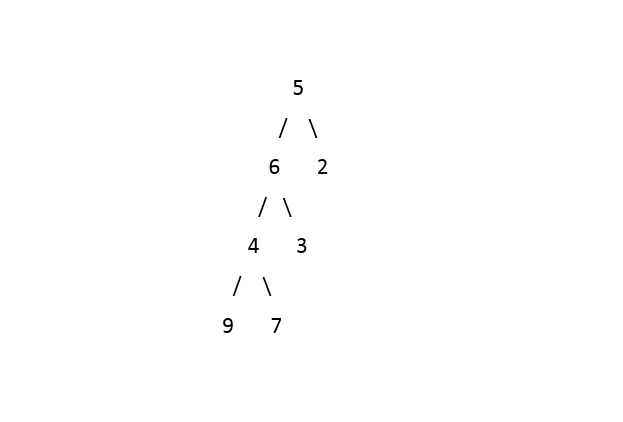1

# Maximum Path Sum Between Two Leaves

Difficulty: HARD
Avg. time to solve
50 min
Success Rate
35%

Problem Statement
Suggest Edit

#### If there is only one leaf node in the tree, then return -1.

##### Note :
``````You do not need to return the path with the maximum sum, just return the maximum sum.
``````
##### Input Format
``````The first line of input contains an integer 'T' representing the number of test cases. Then the test cases follow.

The only line of each test case contains elements in the level order form. The line consists of values of nodes separated by a single space. In case a node is null, we take -1 on its place.

For example, the input for the tree depicted in the below image would be :
````````````1
2 3
4 -1 5 6
-1 7 -1 -1 -1 -1
-1 -1
``````

#### Explanation :

``````Level 1 :
The root node of the tree is 1

Level 2 :
Left child of 1 = 2
Right child of 1 = 3

Level 3 :
Left child of 2 = 4
Right child of 2 = null (-1)
Left child of 3 = 5
Right child of 3 = 6

Level 4 :
Left child of 4 = null (-1)
Right child of 4 = 7
Left child of 5 = null (-1)
Right child of 5 = null (-1)
Left child of 6 = null (-1)
Right child of 6 = null (-1)

Level 5 :
Left child of 7 = null (-1)
Right child of 7 = null (-1)

The first not-null node(of the previous level) is treated as the parent of the first two nodes of the current level. The second not-null node (of the previous level) is treated as the parent node for the next two nodes of the current level and so on.
The input ends when all nodes at the last level are null(-1).
``````
##### Note :
``````The above format was just to provide clarity on how the input is formed for a given tree.
The sequence will be put together in a single line separated by a single space. Hence, for the above-depicted tree, the input will be given as:

1 2 3 4 -1 5 6 -1 7 -1 -1 -1 -1 -1 -1
``````
##### Output Format:
``````For each test case, a single integer representing the maximum path sum between two leaf nodes of the given tree.

The output for each test case is in a separate line.
``````
##### Constraints:
``````1 <= T <= 100
1 <= N <= 5000
0 <= data <= 10^5

Where N is the number of nodes in the tree.
``````
##### Sample Input 1:
``````1
5 6 2 4 3 -1 -1 9 7 -1 -1 -1 -1 -1 -1
``````
##### Sample Output 1:
``````26
``````
##### Explanation of the Sample Input 1:``````The paths between the leaves are as follows :
(1). 9->4->7               (sum = 20)
(2). 9->4->6->3            (sum = 22)
(3). 9->4->6->5->3         (sum = 26)
(4). 7->4->6->3            (sum = 20)
(5). 7->4->6->5->2         (sum = 24)
(6). 3->6->5->2            (sum = 16)

Out of all the above 6 paths, path 9->4->6->5->3 is the maximum sum path with the sum 26.
``````
##### Sample Input 2:
``````1
2 3 -1 -1 -1
``````
##### Sample Output 2:
``````-1
``````
##### Explanation of Sample Input 2:
``````The given tree has only one leaf node (3), thus the answer is -1.
``````